# User:Tohline/Apps/OstrikerBodenheimerLyndenBell66

(Difference between revisions)
Jump to: navigation, search
 Revision as of 09:21, 9 August 2019 (view source)Tohline (Talk | contribs) (→Their Adopted Barotropic Equation of State)← Older edit Revision as of 09:22, 9 August 2019 (view source)Tohline (Talk | contribs) (→Their Adopted Barotropic Equation of State)Newer edit → Line 337: Line 337:
{{User:Tohline/Math/EQ_ZTFG01}} {{User:Tohline/Math/EQ_ZTFG01}} + [https://ui.adsabs.harvard.edu/abs/1966PhRvL..17..816O/abstract OBLB66], p. 817, Eq. (2)

# Rotationally Flattened White Dwarfs

 |   Tiled Menu   |   Tables of Content   |  Banner Video   |  Tohline Home Page   |

## Introduction

As we have reviewed in an accompanying discussion, Chandrasekhar (1935) was the first to construct models of spherically symmetric stars using the barotropic equation of state appropriate for a degenerate electron gas. In so doing, he demonstrated that the maximum mass of an isolated, nonrotating white dwarf is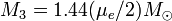$M_3 = 1.44 (\mu_e/2)M_\odot$. A concise derivation of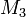$~M_3$ is presented in Chapter XI of Chandrasekhar (1967).

Something catastrophic should happen if mass is greater than$~M_3$. What will rotation do? Presumably it can increase the limiting mass.

 … work by Roxburgh (1965, Z. Astrophys., 62, 134), Anand (1965, Proc. Natl. Acad. Sci. U.S., 54, 23), and James (1964, ApJ, 140, 552) shows that the [Chandrasekhar (1931, ApJ, 74, 81)] mass limit$~M_3$ is increased by only a few percent when uniform rotation is included in the models, … In this Letter we demonstrate that white-dwarf models with masses considerably greater than$~M_3$ are possible if differential rotation is allowed … models are based on the physical assumption of an axially symmetric, completely degenerate, self-gravitating fluid, in which the effects of viscosity, magnetic fields, meridional circulation, and relativistic terms in the hydrodynamical equations have been neglected.

## Solution Strategy

### Our Approach

When the stated objective is to construct steady-state equilibrium models of rotationally flattened, axisymmetric configurations, the accompanying introductory chapter shows how the overarching set of principal governing equations can be reduced in form to the following set of three coupled PDEs (expressed either in terms of cylindrical or spherical coordinates):

Cylindrical Coordinate Base Spherical Coordinate Base

Poisson Equation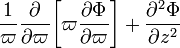$~ \frac{1}{\varpi} \frac{\partial }{\partial\varpi} \biggl[ \varpi \frac{\partial \Phi}{\partial\varpi} \biggr] + \frac{\partial^2 \Phi}{\partial z^2}$$~=$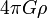$~4\pi G \rho$

The Two Relevant Components of the
Euler Equation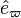$~{\hat{e}}_\varpi$: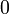$~0$$~=$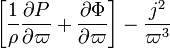$~ \biggl[ \frac{1}{\rho}\frac{\partial P}{\partial\varpi} + \frac{\partial \Phi}{\partial\varpi}\biggr] - \frac{j^2}{\varpi^3}$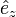$~{\hat{e}}_z$:$~0$$~=$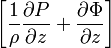$~ \biggl[ \frac{1}{\rho}\frac{\partial P}{\partial z} + \frac{\partial \Phi}{\partial z} \biggr]$

Poisson Equation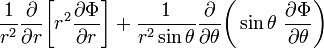$~ \frac{1}{r^2} \frac{\partial }{\partial r} \biggl[ r^2 \frac{\partial \Phi }{\partial r} \biggr] + \frac{1}{r^2 \sin\theta} \frac{\partial }{\partial \theta}\biggl(\sin\theta ~ \frac{\partial \Phi}{\partial\theta}\biggr)$$~=$$~4\pi G\rho$

The Two Relevant Components of the
Euler Equation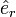$~{\hat{e}}_r$:$~0$ =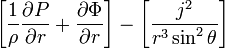$\biggl[ \frac{1}{\rho} \frac{\partial P}{\partial r}+ \frac{\partial \Phi }{\partial r} \biggr] - \biggl[ \frac{j^2}{r^3 \sin^2\theta} \biggr]$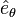$~{\hat{e}}_\theta$:$~0$ =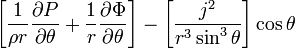$\biggl[ \frac{1}{\rho r} \frac{\partial P}{\partial\theta} + \frac{1}{r} \frac{\partial \Phi}{\partial\theta} \biggr] - \biggl[ \frac{j^2}{r^3 \sin^3\theta} \biggr] \cos\theta$

This set of simplified governing relations must then be supplemented by a specification of: (a) a barotropic equation of state,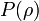$~P(\rho)$; and (b) the equilibrium configurations's radial specific angular momentum profile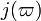$~j(\varpi)$. How does this recommended modeling approach compare to the approach outlined by Ostriker, Bodenheimer & Lynden-Bell (1966?

### Approach Outlined by Ostriker, Bodenheimer & Lynden-Bell (1966)

#### Their Equation (4)

One can immediately appreciate that, independent of the chosen coordinate base, the first expression listed among our trio of governing PDEs derives from the differential representation of the Poisson equation as discussed elsewhere and as has been reprinted here as Table 1.

Table 1:  Poisson Equation
Integral Representation Differential Representation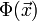$~ \Phi(\vec{x})$$~=$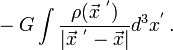$~ -G \int \frac{\rho(\vec{x}^{~'})}{|\vec{x}^{~'} - \vec{x}|} d^3x^' \, .$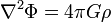$\nabla^2 \Phi = 4\pi G \rho$

Ostriker, Bodenheimer & Lynden-Bell (1966; hereafter, OBLB66) chose, instead, to use the integral representation of the Poisson equation to evaluate the gravitational potential; specifically, they write,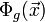$~ \Phi_g(\vec{x})$$~=$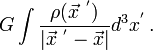$~ G \int \frac{\rho(\vec{x}^{~'})}{|\vec{x}^{~'} - \vec{x}|} d^3x^' \, .$ OBLB66, p. 817, Eq. (4)

(Note that, in defining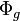$~\Phi_g$, OBLB66 have adopted a sign convention for the gravitational potential that is the opposite of ours; that is,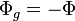$~\Phi_g = - \Phi$.)

#### Their Equations (3) & (5)

The two relevant components of the Euler equation that are identified, above, result from imposing a steady-state condition on the,

Eulerian Representation
of the Euler Equation,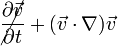$~\cancel{\frac{\partial \vec{v}}{\partial t} } + (\vec{v} \cdot \nabla)\vec{v}$$~=$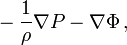$~ - \frac{1}{\rho} \nabla P - \nabla \Phi \, ,$

and adopting a steady-state rotational velocity field in which the angular velocity is either constant or is only a function of the cylindrical-coordinate radius,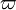$~\varpi$; that is,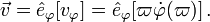$~\vec{v} = \hat{e}_\varphi [v_\varphi] = \hat{e}_\varphi [\varpi \dot\varphi (\varpi)] \, .$

As we have demonstrated in an accompanying discussion, for any of a number of astrophysically relevant simple rotation profiles of this form, the convective operator on the left-hand side of this steady-state Euler equation gives (most conveniently written here in a cylindrical-coordinate base),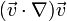$~(\vec{v} \cdot \nabla)\vec{v}$$~=$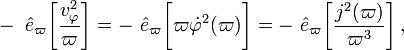$~-~\hat{e}_\varpi \biggl[\frac{v_\varphi^2}{\varpi} \biggr] = -~\hat{e}_\varpi \biggl[ \varpi {\dot\varphi}^2(\varpi) \biggr] = -~\hat{e}_\varpi \biggl[\frac{j^2(\varpi)}{\varpi^3} \biggr] \, ,$

where,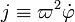$~j \equiv \varpi^2 \dot\varphi$ is the (radially dependent) specific angular momentum measured relative to the symmetry (rotation) axis. As we have pointed out in an accompanying discussion, this last expression can be rewritten in terms of the gradient of a scalar (centrifugal) potential; specifically,$~(\vec{v} \cdot \nabla) \vec{v}$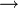$~\rightarrow$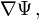$~\nabla \Psi \, ,$

if the centrifugal potential is defined such that,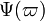$~\Psi(\varpi)$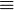$~\equiv$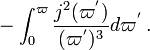$~- \int_0^\varpi \frac{j^2(\varpi^')}{(\varpi^')^3} d\varpi^' \, .$ OBLB66, p. 817, Eq. (5)

(Note that OBLB66 adopted a sign convention for the centrifugal potential that is the opposite of ours; that is,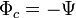$~\Phi_c = - \Psi$.) Hence, assuming that our intent is to construct a rotationally flattened equilibrium configuration whose rotation profile is of the form,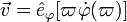$~\vec{v} = \hat{e}_\varphi [\varpi \dot\varphi (\varpi)]$, the steady-state Euler equation can be rewritten as,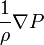$~\frac{1}{\rho} \nabla P$$~=$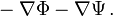$~ - \nabla \Phi - \nabla \Psi \, .$ OBLB66, p. 817, Eq. (3)

#### Their Adopted Angular-Momentum Distribution

In what follows, text that has been extracted directly from p. 817 of OBLB66 is presented using a dark green font.

"The angular-velocity distribution in the model is determined through the specification of a distribution of angular momentum per unit mass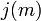$~j(m)$, where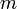$~m$ is a Lagrangian coordinate equal to the fraction of the total mass interior to a cylindrical surface around the axis of rotation. The specification of$~j(m)$ rather than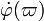$~\dot\varphi(\varpi)$ permits the construction of equilibrium models for a given choice of [total] angular momentum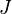$~J$. The angular-momentum distribution chosen for the computed models is that of a uniformly rotating polytrope of index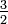$~\tfrac{3}{2}$."

Later papers refer to models with OBLB66's specified angular momentum profile as belonging to an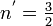$~n^' = \tfrac{3}{2}$ sequence. It cannot be described by a closed-form analytic expression. But, as a point of reference and drawing from Stoeckly's (1965) work, in an accompanying discussion we derive the analytic expression for the angular momentum distribution of models that lie along a so-called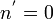$~n^' = 0$ sequence.

#### Their Adopted Barotropic Equation of State

Because they were interested in constructing equilibrium models of rotationally flattened white dwarfs, OBLB66 chose a barotropic equation of state that describes a zero-temperature Fermi (degenerate electron) gas. As we have documented in our accompanying discussion of barotropic equations of state, the set of key relations that define this equation of state is,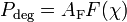$~P_\mathrm{deg} = A_\mathrm{F} F(\chi)$ where: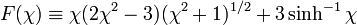$F(\chi) \equiv \chi(2\chi^2 - 3)(\chi^2 + 1)^{1/2} + 3\sinh^{-1}\chi$ and: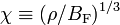$\chi \equiv (\rho/B_\mathrm{F})^{1/3}$

OBLB66, p. 817, Eq. (2)

# See Also

 © 2014 - 2019 by Joel E. Tohline |   H_Book Home   |   YouTube   | Context: | PGE | SR | Appendices: | Equations | Variables | References | Binary Polytropes | Ramblings | Images | Images (2016 Layout) | ADS |